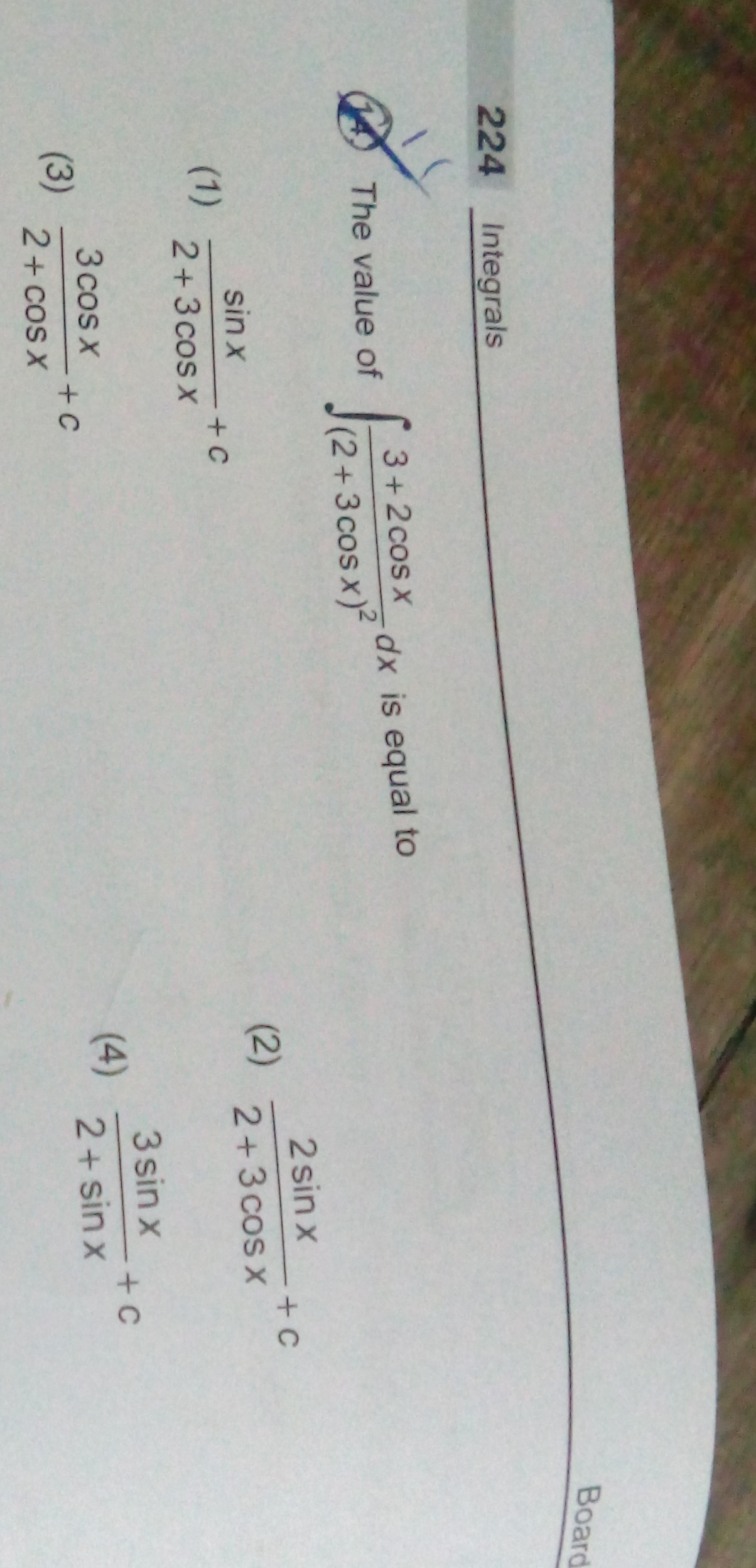## Guest4 years ago
Dear Amit

∫ 3 + 2 cos x / (2+ 3 cos x)2 dx Let I =

Multiplying Nr. & Dr. by cosec2x

=> I = int {{(3cosec^{2}x+ 2cot x cosec x) } / (2cosec x +3cot x)^2  }dx

= - int {{(-3cosec^{2}x- 2cot x cosec x) } / (2cosec x +3cot x)^2  }dx

Let us consider 2cosec x +3cot x=t

then    dt = (-3cosec^{2}x- 2cot x cosec x).dx

=int (dt / t^2)

integrate it then we get

={1} / {(2cosec x +3cot x)}

={sin x}  / {2+3cos x} +c